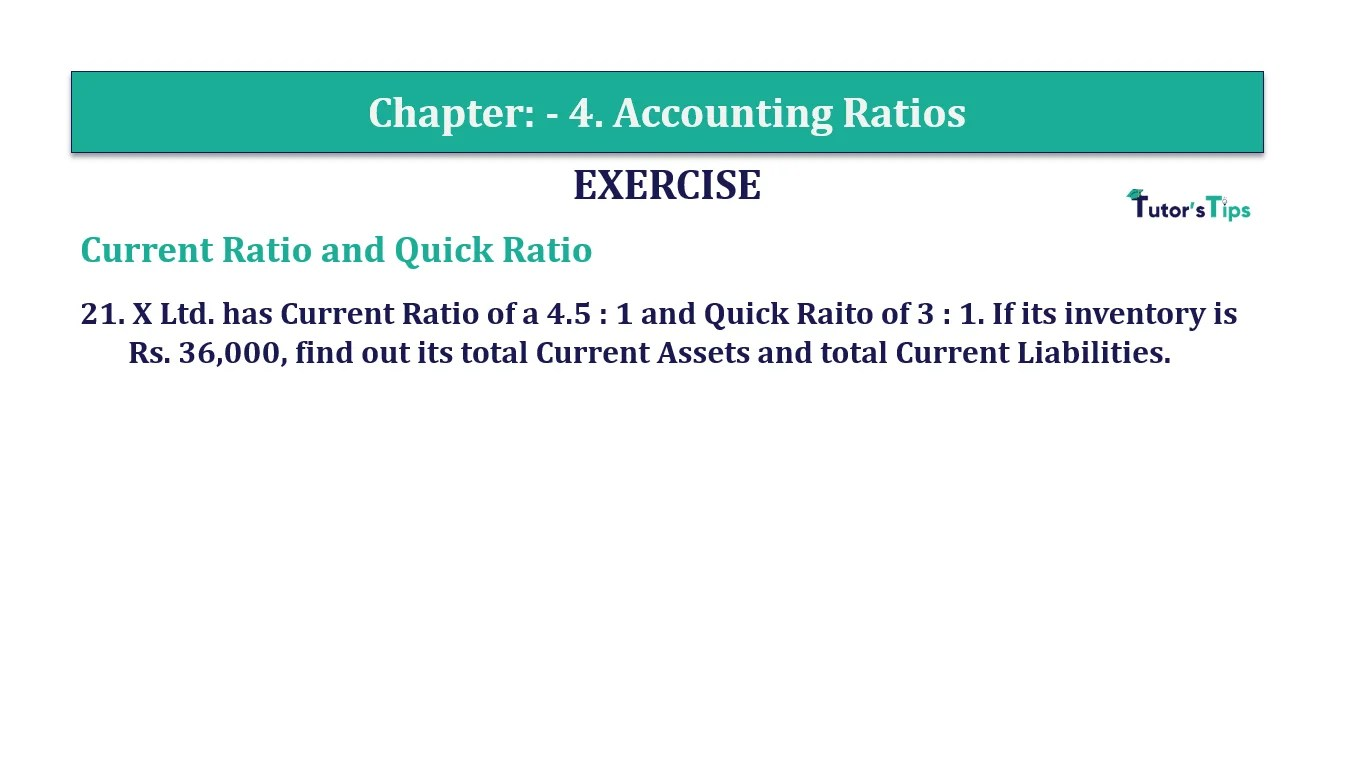# Question 21 Chapter 4 of +2-B – T.S. Grewal 12 ClassQuestion No. 21 - Chapter No.4 - T.S. Grewal +2 Book Part B

Question 21 Chapter 4 of +2-B

Current Ratio and Quick Ratio

21. X Ltd. has Current Ratio of a 4.5: 1 and Quick Raito of 3: 1. If its inventory is Rs. 36,000, find out its total Current Assets and Total Current Liabilities.

### The solution of Question 21 Chapter 4 of +2-B: –

 Current Ratio = Current Assets = 4.5 Current Liabilities 1

 Quick Ratio = Quick Assets = 3 Current Liabilities 1

 Inventories = 36,000 Let Current Liabilities = x Current Assets = 4.5x Quick Assets = 3x Inventories = Current Assets – Quick Assets Rs. 36,000 = 4.5x – 3x Rs. 36,000 = 1.5x x = Rs. 24,000 Current Liabilities = x = Rs. 24,000

 Current Assets = 4.5 x Rs.24,000 = Rs. 1,08,000

 Quick Assets = 3 x Rs. 24,000 = Rs. 72,000

Balance Sheet: Meaning, Format & Examples

Comment if you have any question.

Also, Check out the solved question of previous Chapters: –